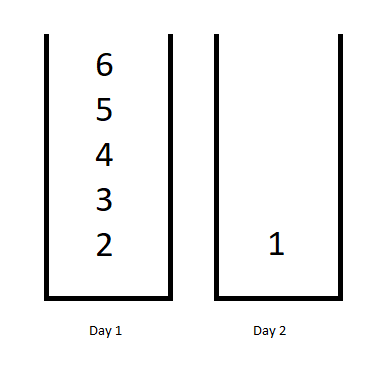# 410. 分割数组的最大值# 1143. Longest Common Subsequence

Given two strings text1 and text2, return common subsequence. If there is no common subsequence, return 0.# 1335. Minimum Difficulty of a Job Schedule

You want to schedule a list of jobs in d days. Jobs are dependent (i.e To work on the i-th job, you have to finish all the jobs j where 0 <= j < i).# Dynamic Programming - 375. Guess Number Higher or Lower II

375. Guess Number Higher or Lower II We are playing the Guess Game. The game is as follows: I pick a number from 1 to n. You have to guess which number I picked. Every time you guess wrong, I’ll tell you whether the number I picked is higher or lower. However, when you guess a particular number x, and you guess wrong, you pay \$x. You win the game when you guess the number I picked. Example: n = 10, I pick 8. First round: You guess 5, I tell you that it's higher. You pay ....# Dynamic Programming - 377. Combination Sum IV

377. Combination Sum IV Given an integer array with all positive numbers and no duplicates, find the number of possible combinations that add up to a positive integer target. Example: nums_ = [1, 2, 3] target = 4 The possible combination ways are: (1, 1, 1, 1) (1, 1, 2) (1, 2, 1) (1, 3) (2, 1, 1) (2, 2) (3, 1) Note that different sequences are counted as different combinations. Therefore the output is 7. Follow up: What if negative numbers are allowed in the given array? How does it change....

Nothing just happens, it's all part of a plan.